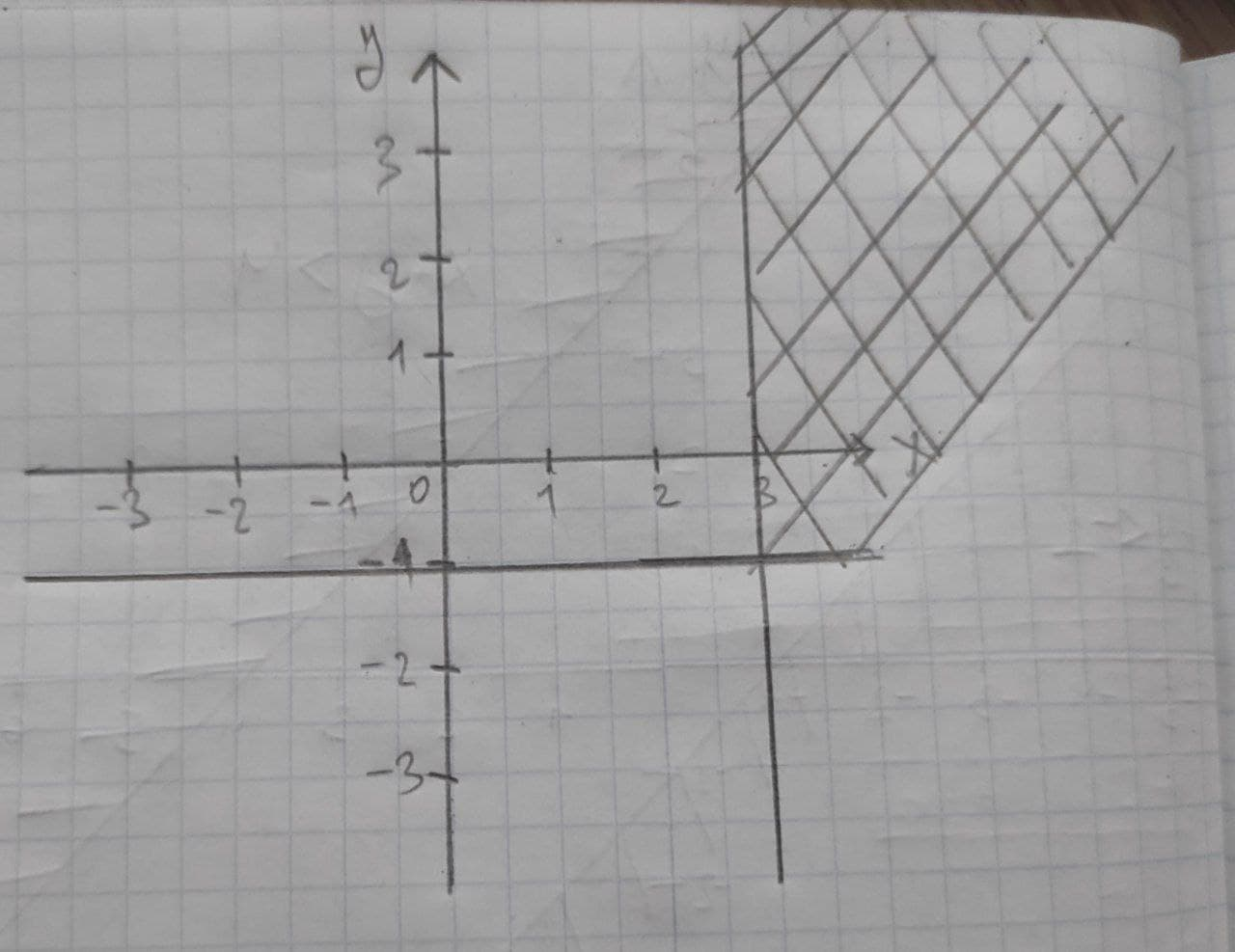# Graph the solution set for the system of linear inequalities x>=3 y>=-1Maiclubk 2020-11-05 Answered
Graph the solution set for the system of linear inequalities
$x\ge 3$
$y\ge -1$
You can still ask an expert for help

## Want to know more about Inequalities systems and graphs?

• Questions are typically answered in as fast as 30 minutes

Solve your problem for the price of one coffee

• Math expert for every subject
• Pay only if we can solve itopsadnojD

Auxiliary equations are x=3 and y=-1
We graph those lines first.
x=3 will be a vertical line passing through x=3
And y=-1 is a horizontal line passing through y=-1
For $x\ge 3$ shade the right side of x=3
For $y\ge -1$ we shade above y=-1.